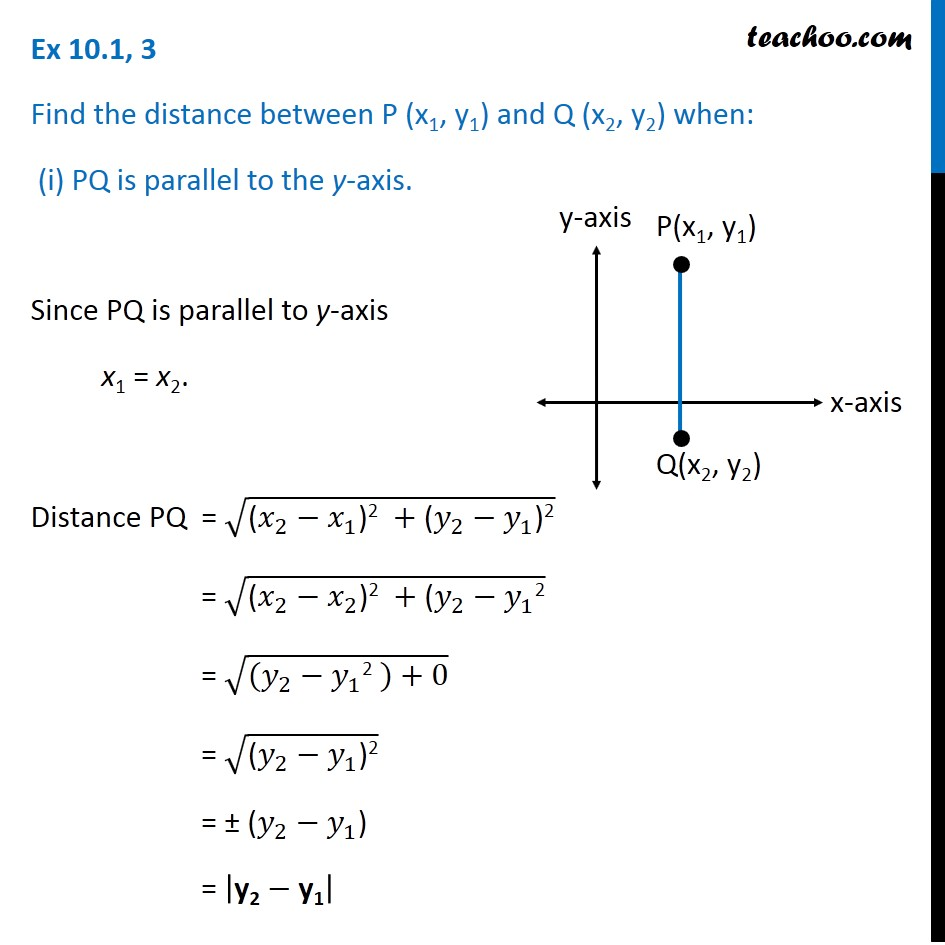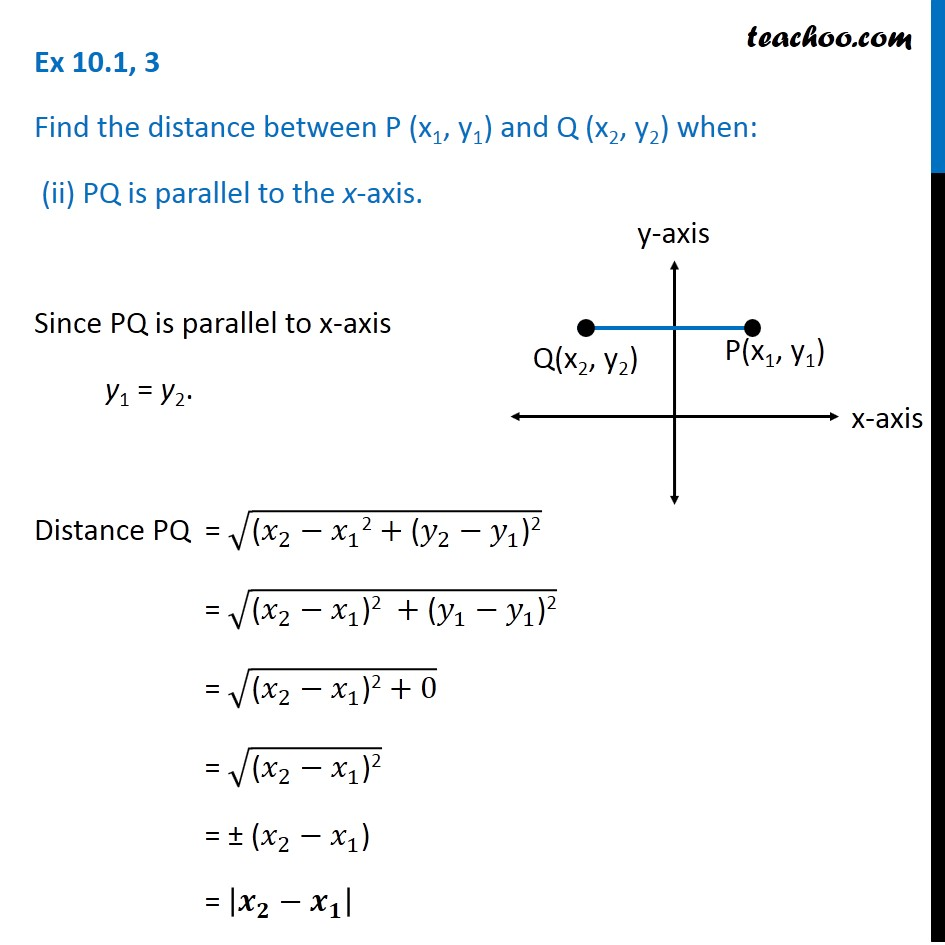Subscribe to our Youtube Channel - https://you.tube/teachoo

1. Chapter 10 Class 11 Straight Lines
2. Serial order wise
3. Ex 10.1

Transcript

Ex 10.1, 3 Find the distance between P (x1, y1) and Q (x2, y2) when: (i) PQ is parallel to the y-axis. Since PQ is parallel to y-axis x1 = x2. Distance PQ = √("(" 𝑥_2−𝑥_1 ")2 " +"(" 𝑦_2−𝑦_1 ")2" ) = √("(" 𝑥_2−𝑥_2 ")2 " +"(" 𝑦_2−𝑦_1 "2" ) = √((𝑦_2−𝑦_1 "2" )+0) = √("(" 𝑦_2−𝑦_1 ")2" ) = ± (𝑦_2−𝑦_1) = |"y2" −"y1" | Ex 10.1, 3 Find the distance between P (x1, y1) and Q (x2, y2) when: (ii) PQ is parallel to the x-axis. Since PQ is parallel to x-axis y1 = y2. Distance PQ = √("(" 𝑥_2−𝑥_1 "2" +"(" 𝑦_2−𝑦_1 ")2" ) = √("(" 𝑥_2−𝑥_1 ")2 " +"(" 𝑦_1−𝑦_1 ")2" ) = √("(" 𝑥_2−𝑥_1 ")2" +0) = √("(" 𝑥_2−𝑥_1 ")2" ) = ± (𝑥_2−𝑥_1) = |𝒙_𝟐−𝒙_𝟏 |

Ex 10.1